# Printable Multiplication WorksheetsUpdated on Feb 02, 2023By Printablee Team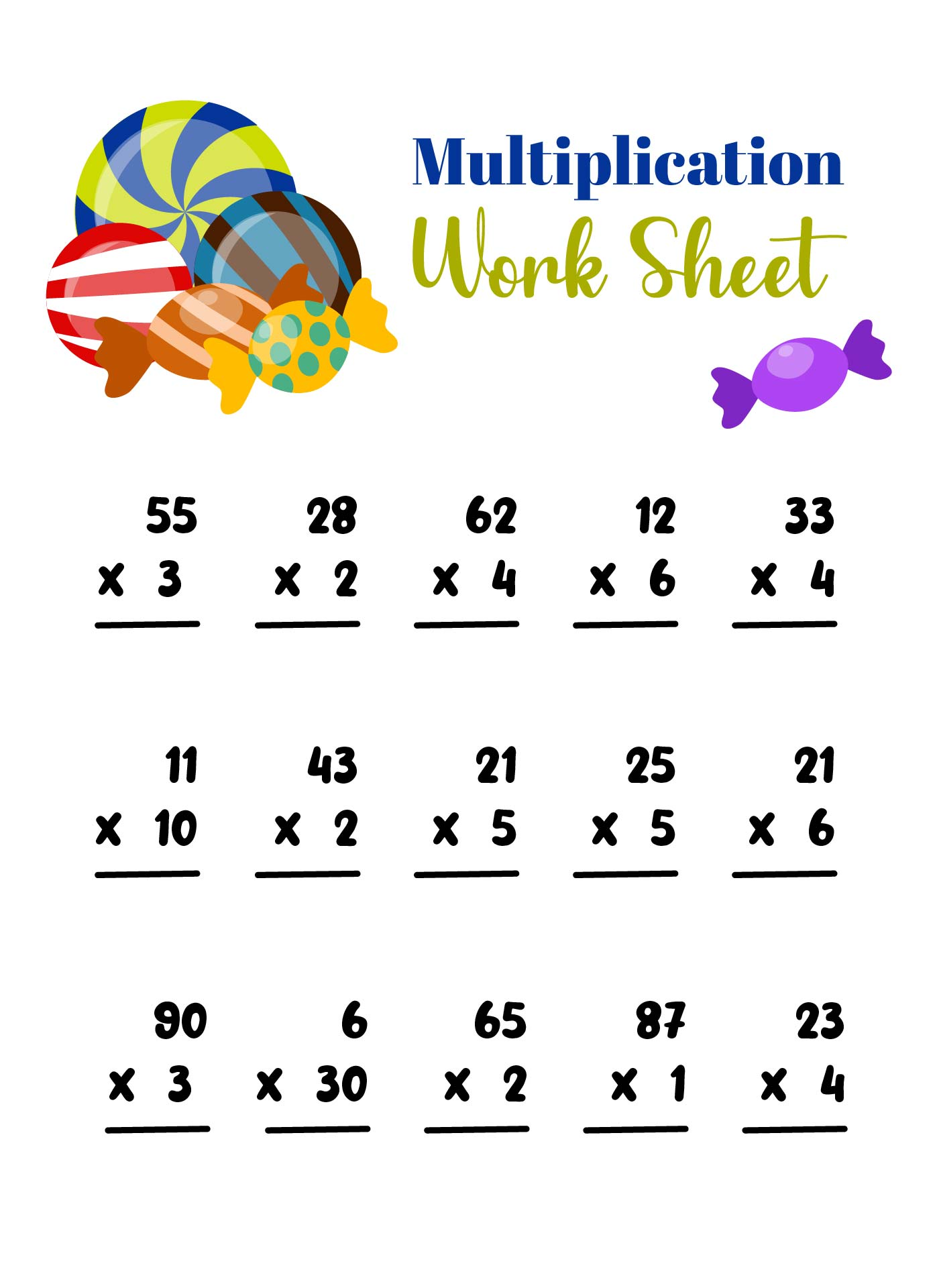## What is Multiplication?

Multiplication worksheets are widely used by teachers and parents to make it easier for children to learn how to multiply values. The reason is, this is indeed something that is quite difficult for children to do.

So, before discussing the subject further, let's discuss its definition of it first. It is one of the four basic arithmetic operations such as division, subtraction, and addition.

Multiplication itself has the concept of repeated addition of a group of the same number. To make things clearer, let's look at an example first.

For example, in one box there are two bags. Each bag contains 3 candies. If you add them up, the total candy in the box is 3 + 3 = 6. Now, because every two bags has the same number of candies, so you can multiply them.

Because there are 2 bags and each bag contains 3 candies, the multiplication becomes 2 x 3 = 6. So, the result is the same as the sum, right?

In the 2 x 3 multiplication concept, the number 2 is called the multiplier and the number 3 is the multiplicand. Meanwhile, 6 is the multiplication product.

The symbol for multiplication itself uses a cross (x). However, you can also use an asterisk (*). The asterisk is usually used to provide its symbols when programming or typing on computers and also algebra.

## Easy Guide to Multiplying Factors for Kids

Learning math as with many other basic concepts is an important thing to do. The reason is, the higher the level of a child's school, the more this kind of product of numbers will be needed. Because it is very important, they must be taught from an early age for a better understanding.

However, children may find it difficult with multiplication so they get confused when they have to complete the assignment given. Don't worry, we provide 3 easy guides so children can easily learn and fill in their math tasks!

1. ### Turn Multiplication into Addition

Many parents and teachers teach this basic math to children by asking children to fix it inside their minds. In fact, this method is not always effective. Why? The reason is, children can forget multiplication if they just memorize it.

Therefore, compared to asking children to memorize, it's better to explain to them in an easy way. An example is as we explained before, namely by changing multiplication into addition.

You can add pictures to support their understanding of the concept. In this way, children will slowly be able to understand the concept without having to memorize it.

2. ### Start Ahead with the Multiplication of Zero and One

Don't rush to teach your child multiplication with large numbers. Start with zero and one. Explain to the children that adding a zero has no effect on the sum of the numbers. Well, after that, explain to them that multiplying any number by zero will always produce zero.

Once the children have grasped the concept, move on to teaching them that multiplying any number by one will still produce the same number.

3. ### Continue with Bigger Numbers, but Still Easy

In studying and filling them out, a multiplication table is still needed to make it easier for children. Now, after teaching the two previous concepts, test children regarding these two concepts. Don't forget to cover the multiplication table so your child doesn't copy it.

After the children understand these two concepts, continue teaching them about multiplication with large but still easy numbers. An example is 10 x 10. They also need to teach about using charts by multiplying numbers in horizontal and vertical positions.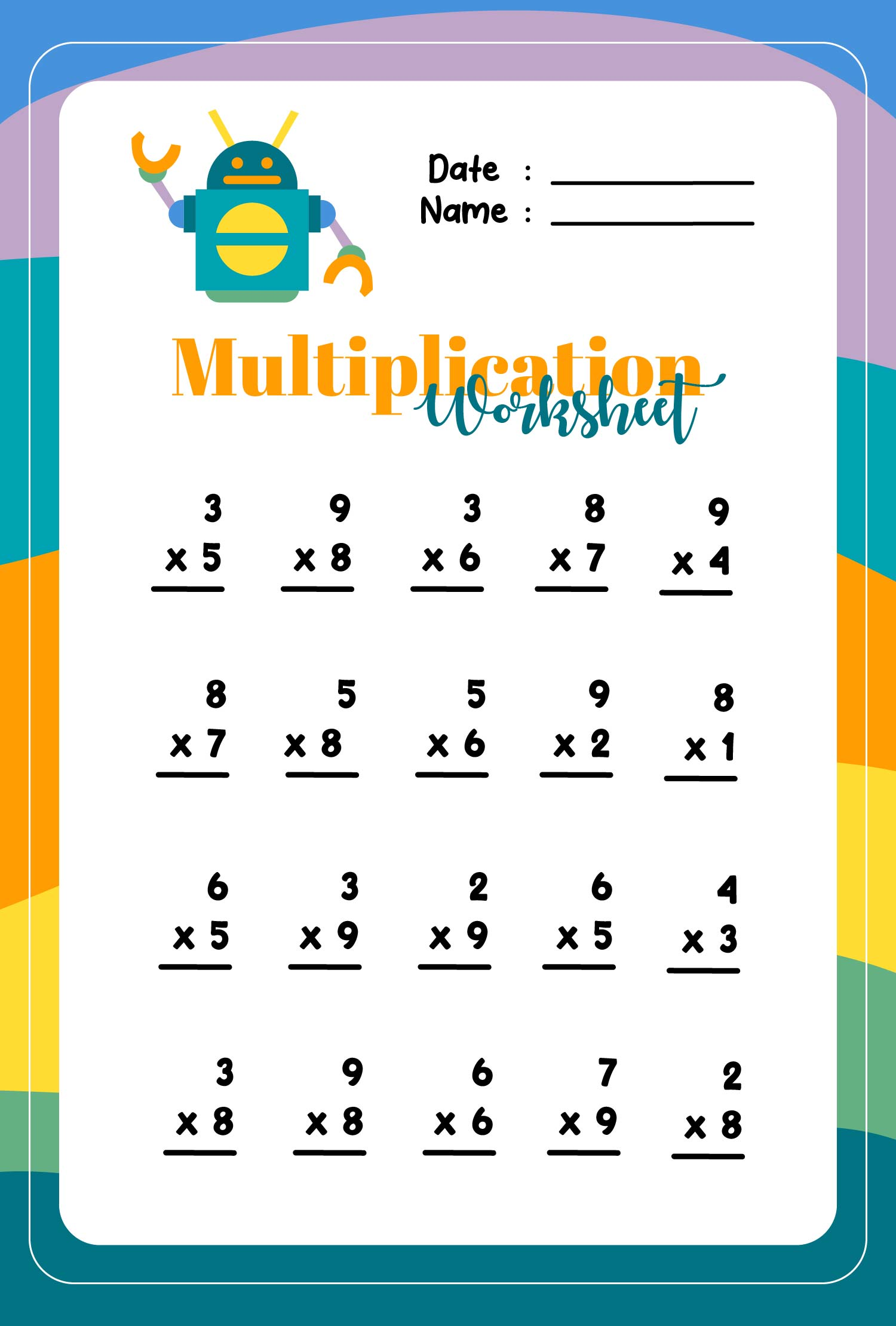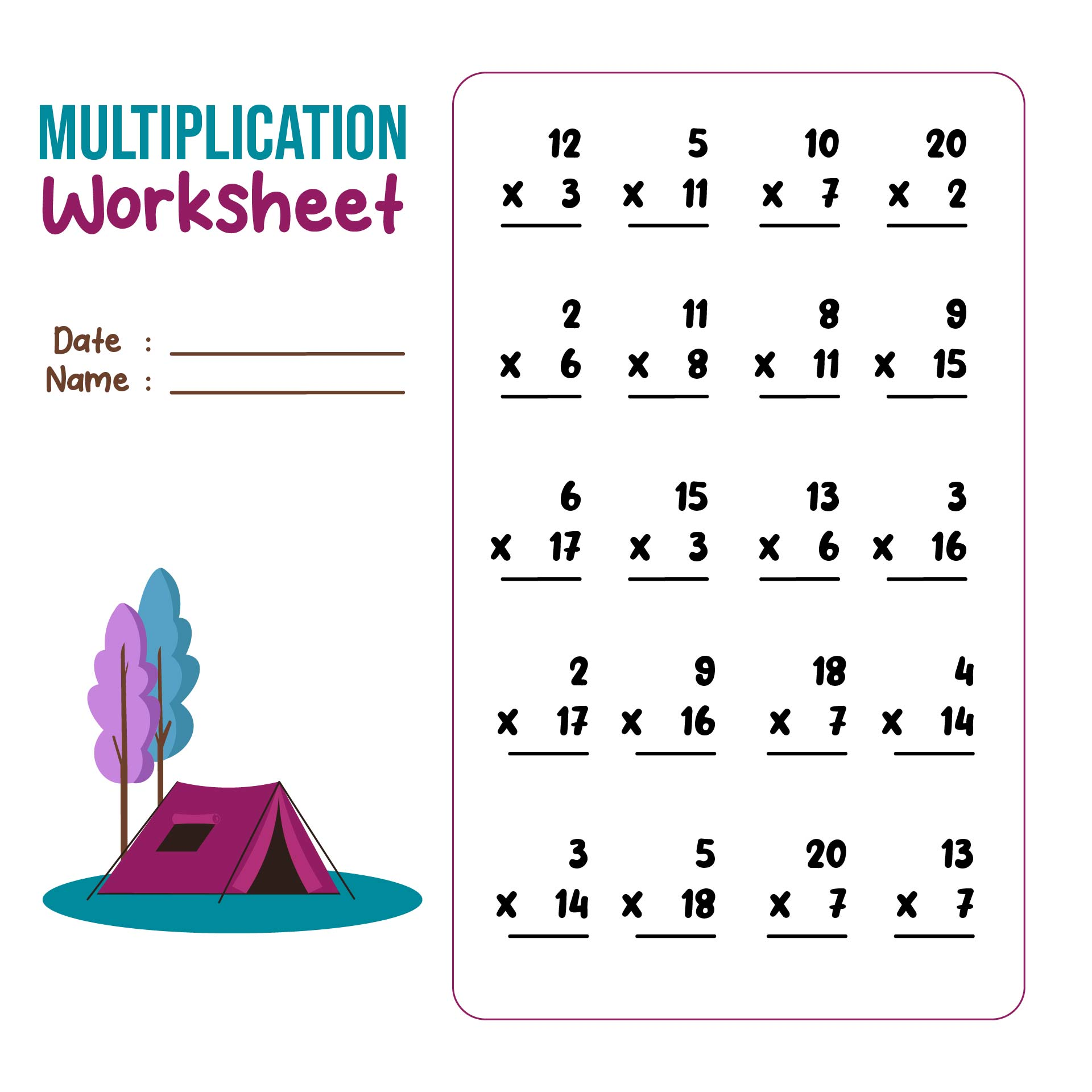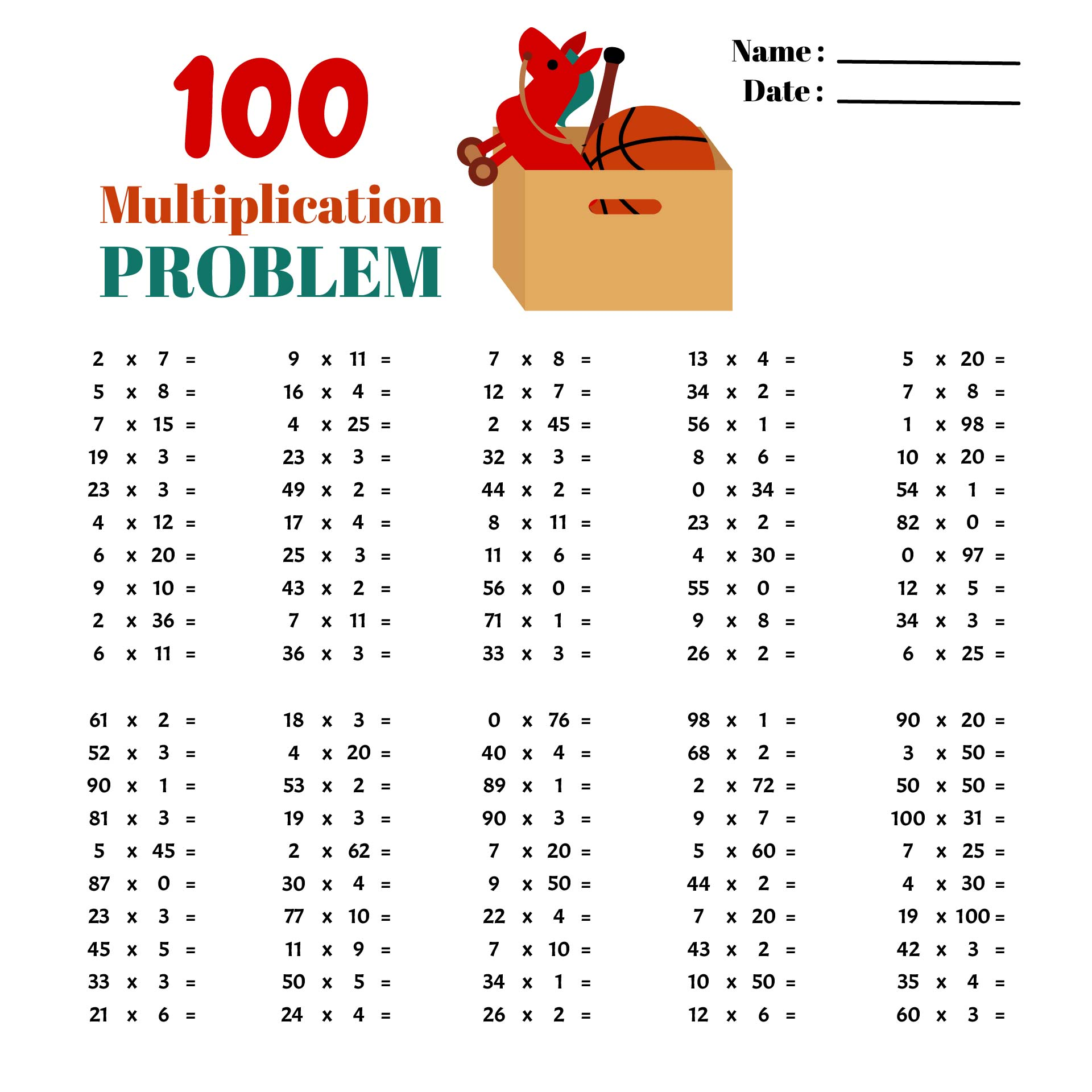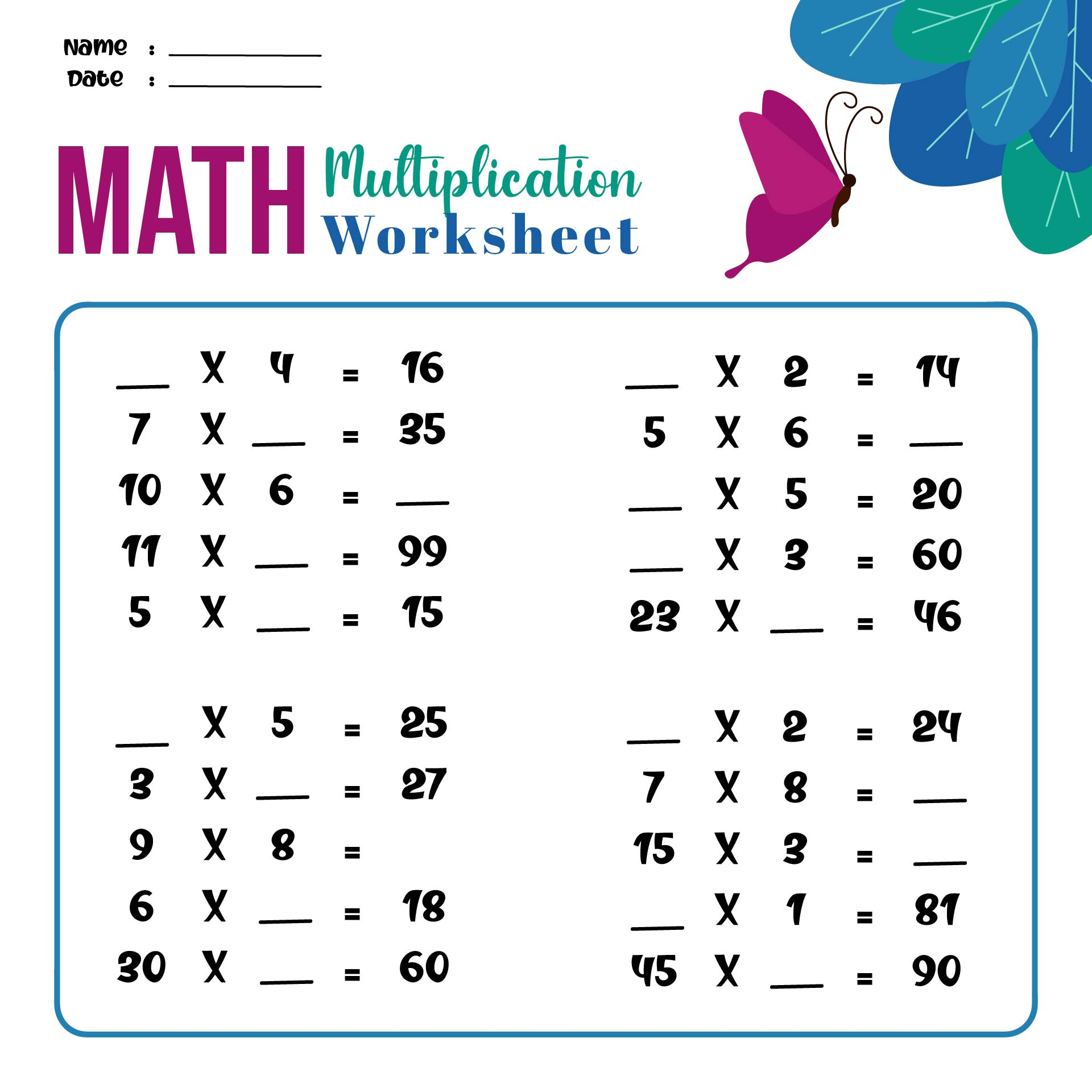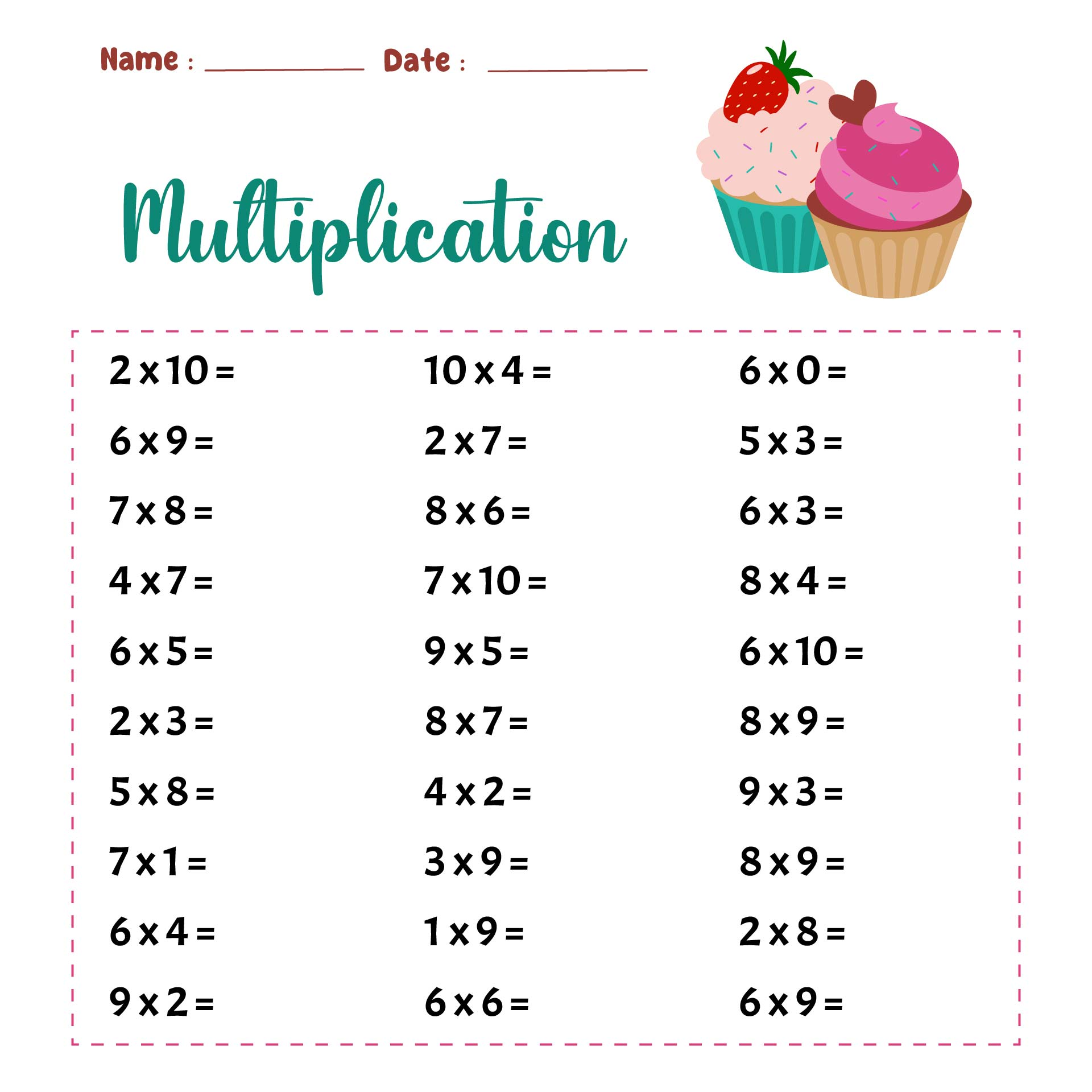## What are The Practical Benefits of Learning to Multiply Numbers for Children?

There are many kinds of multiplication problem practice that you can find here. So, you don't have to worry about looking for the suitable one for your kids or students.

This will make it easier for you to train children regarding multiplication. Not only is easy, but this kind of task is also flexible because it can be used anywhere and anytime. This can be a great tool for educators to teach level Kindergarten and 1st to 5th graders.

By doing and learning through these assignments, there are several benefits that can be obtained from children.

1. ### Develop Time Management Ability

Most children do not have good time management when working on math problems. Therefore, by completing the challenge on task, they will get used to doing math problems in a timely manner.

Why? The reason is while working on the worksheet, children must be able to complete it within the allotted time. So, if the child does it regularly, it is certain that after a while the child will be able to do math problems quickly.

2. ### Train Children's Focus

Multiplication Worksheets require high focus. By studying this basic math, children can concentrate more on doing anything. So, not only will it make children able to understand the concept of multiplication, but can also teach children to be more focused.
3. ### Good Critical Thinking

By diligently practicing, children will be able to have good problem-solving skills. The reason is that this requires high accuracy and understanding, so children can be trained to think more critically.

That way, it becomes easier for children to work on various problems or other problems they encounter. This method will eventually bring up children's self-confidence because they feel they can solve problems easily.